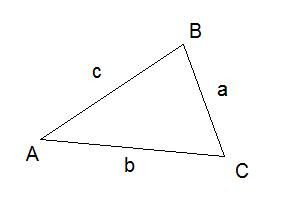# Trigonometry - Sine Law (Law of Sines)

The trig functions that I've discussed so far (Sine, Cosine, and Tangent) will be incredibly useful to you when working specifically with right angle triangles. However, of course, not all triangles have a 90 degree angle in them. So can you still use these functions? Well, yes, but in a different way. One way is through application of the Law of Sines.

Let's consider a triangle that has three different angles, none of which are right angles. The standard naming scheme still applies, although now there is no hypotenuse (remember, the hypotenuse is opposite to the 90 degree angle).But what is sine law?  The trigonometry sine law says that the ratio between the sine of an angle and the side opposite to it will be equal for all three angles. In other words:

a/SinA = b/SinB = c/SinC

Since you only really work with 2 ratios at a time, with a little rearranging, you can see that:

aSinB = bSinA

So let's try some sine law examples. Say we have a triangle with 1 known angle of 40 degrees, sides 4 and 6 units long. Find angle B:The Sine Law says that the ratios of angle to opposite sides will be equal. With this, we know how to find an angle using sine law.  So we have:

6 x (Sin 40) = 4 x (SinB)
SinB = 0.964
B = 74.62 degrees

Now, if the question had asked to find all the angles in this triangle, you can simply say that since the 3 angles in the triangle will add up to 180, you can just subtract your known angles from 180 to get the third angle:

180 = 40 + 74.62 + C
C = 65.38

And now that you have angle C, you can use the Law of Sines with that angle to solve for the final unknown side:

cSinA = aSinC
c x (Sin 40) = 4 x (Sin 65.38)
c = 5.66

Whenever you are given a triangle that does not include a right angle, but you are provided with 1) an angle, 2) the length of the side opposite to that angle, and 3) any one of either the other sides or angles, then you can use the Sine Law.  Now you know how to find an angle using sine law!

#### 10 comments:

1.thanks..... that helped me with my math hw!!!!!!!

2.you dont know how much thats helped!!!

3.I did poorly trig when I took it in high school because the teacher was a jerk and I didn't see a point in learning it.

Now I'm relearning it because I'm studying animation with shapes in actionscript (flash) for web development. Thanks for the tutorial.

4.So, if the known angle was 50 degrees, why did the problem show 40 degrees? I don't get it.

5.Mick,

Sorry about the error. I've corrected the problem now. It should have been 40 in the text, not 50.

6.wat if, if the 40 degrees is in the angle C?

7.If angle C were 40 degrees (instead of angle A), then the sine law doesn't apply anymore. Remember you need one complete set of side + angle opposite to that side, which you wouldn't have. However, since you would instead have 2 sides and the angle created by those 2 sides, you can you the cosine rule instead to solve the last side, and then start applying the sine rule to find the last angles.

8.hey I did not understand how SinB = 0.964 because when I plug in my calculator 6*(Sin40) it did not come out to be 0.964

9.You need to isolate the sinB... ie, divide the 6*sin40 by 4.

10.THANKYOU VERY MUCH!!!
IT REALLY HELPED ME..
THNX AGAIN-
GAV